#### Feasibility and optimality

The notions of feasible and optimal plans need to be reconsidered in the context of feedback planning because the initial condition is not given. A plan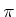is called a solution to the feasible planning problem if from every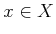from which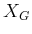is reachable the goal set is indeed reached by executingfrom. This means that the cost functional is ignored (an alternative to Formulation 8.1 can be defined in which the cost functional is removed). For convenience,will be called a feasible feedback plan.

Now consider optimality. From a given state, it is clear that an optimal plan exists using the concepts of Section 2.3. Is it possible that a different optimal plan needs to be associated with everythat can reach? It turns out that only one plan is needed to encode optimal paths from every initial state to. Why is this true? Suppose that the optimal cost-to-go is computed overusing Dijkstra's algorithm or value iteration, as covered in Section 2.3. Every cost-to-go value at someindicates the cost received under the implementation of the optimal open-loop plan from. The first step in this optimal plan can be determined by (2.19), which yields a new state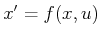. From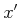, (2.19) can be applied once again to determine the next optimal action. The cost atrepresents both the optimal cost-to-go ifis the initial condition and also the optimal cost-to-go when continuing on the optimal path from. The two must be equivalent because of the dynamic programming principle. Since all such costs must coincide, a single feedback plan can be used to obtain the optimal cost-to-go from every initial condition.

A feedback planis therefore defined as optimal if from every, the total cost,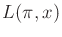, obtained by executingis the lowest among all possible plans. The requirement that this holds for every initial condition is important for feedback planning.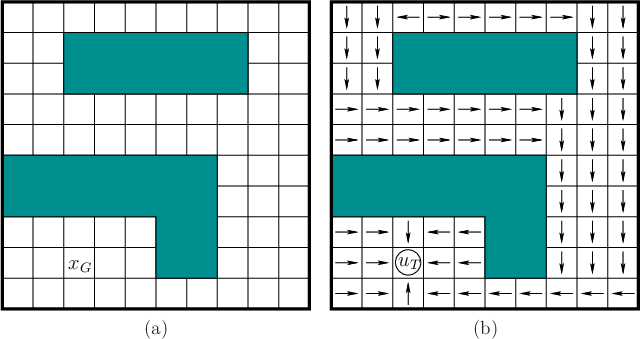Example 8..1 (Feedback Plan on a 2D Grid)   This example uses the 2D grid model explained in Example 2.1. A robot moves on a grid, and the possible actions are up (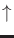), down (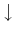), left (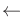), right (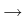), and terminate (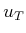); some directions are not available from some states. A solution feedback plan is depicted in Figure 8.2. Many other possible solutions plans exist. The one shown here happens to be optimal in terms of the number of steps to the goal. Some alternative feedback plans are also optimal (figure out which arrows can be changed). To apply the plan from any initial state, simply follow the arrows to the goal. In each stage, the application of the action represented by the arrow leads to the next state. The process terminates whenis applied at the goal.Steven M LaValle 2020-08-14